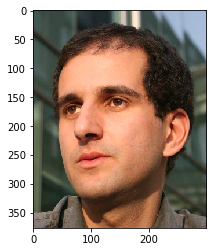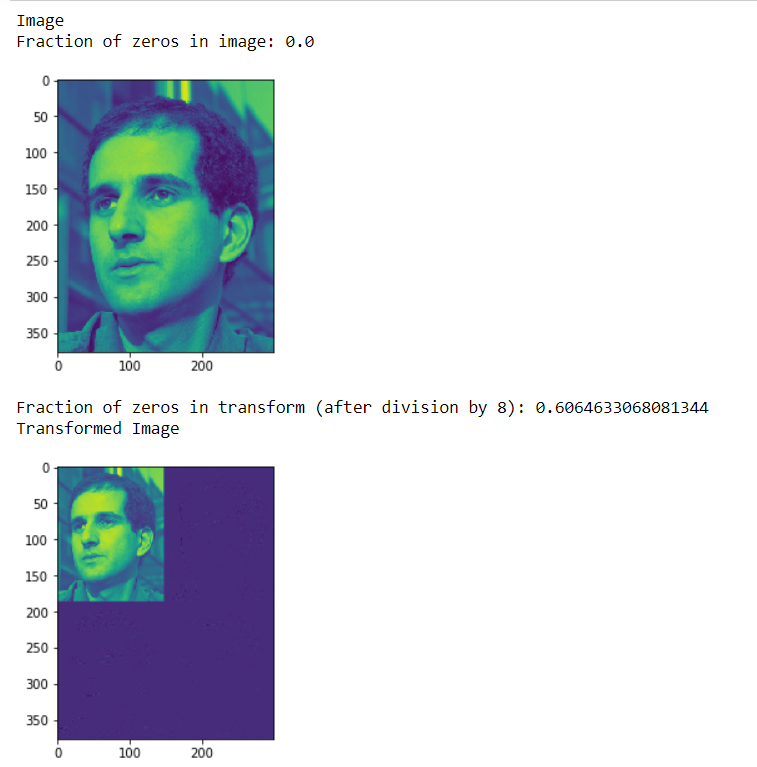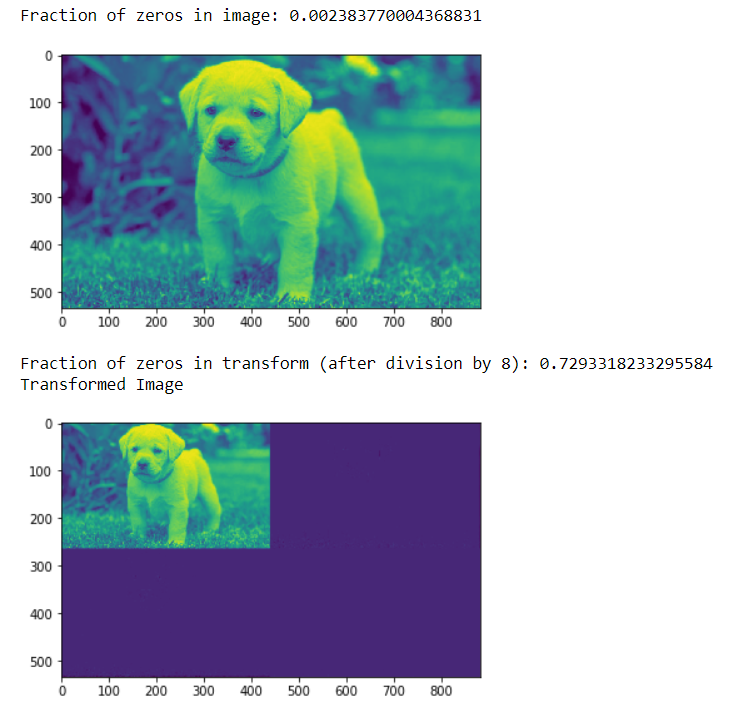# Mahotas – Fraction of zeros in image

In this article we will see how we can get the fraction of zeros in the image in mahotas. Fraction of zeros is the percentage amount of statistical data which is zero. It is relevant in statistical models where a significant amount of objects has zero value.

In this tutorial we will use “luispedro” image, below is the command to load it.

`mahotas.demos.load('luispedro')`

Below is the luispedro imageIn order to do this we will use `np.mean` method

Syntax : np.mean(img==0)

Argument : It takes image object as argument

Return : It returns numpy.float64

Note : Input image should be filtered or should be loaded as grey

In order to filter the image we will take the image object which is numpy.ndarray and filter it with the help of indexing, below is the command to do this

`image = image[:, :, 0]`

Example 1:

 `# importing various libraries ` `import` `numpy as np ` `import` `mahotas ` `import` `mahotas.demos ` `from` `mahotas.thresholding ``import` `soft_threshold ` `from` `pylab ``import` `imshow, show ` `from` `os ``import` `path ` ` `  `# loading image ` `f ``=` `mahotas.demos.load(``'luispedro'``, as_grey ``=` `True``) ` ` `  ` `  `# showing image ` `print``(``"Image"``) ` ` `  `# getting fraction of zeros in image ` `fraction ``=` `np.mean(f ``=``=` `0``) ` ` `  `print``(``"Fraction of zeros in image: {0}"``.``format``(fraction)) ` `imshow(f) ` `show() ` ` `  ` `  ` `  `# Transform using D8 Wavelet to obtain transformed image t ` `t ``=` `mahotas.daubechies(f, ``'D8'``) ` ` `  `# Discard low-order bits: ` `t ``/``=` `8` `t ``=` `t.astype(np.int8) ` ` `  ` `  `# getting fraction of zeros in image ` `fraction ``=` `np.mean(t ``=``=` `0``) ` ` `  `print``(``"Fraction of zeros in transform (after division by 8): {0}"``.``format``(fraction)) ` ` `  `# showing transformed image ` `print``(``"Transformed Image"``) ` `imshow(t) ` `show() `

Output :Example 2:

 `# importing required libraries ` `import` `mahotas ` `import` `numpy as np ` `from` `pylab ``import` `imshow, show ` `import` `os ` ` `  ` `  `# loading iamge ` `img ``=` `mahotas.imread(``'dog_image.png'``) ` ` `  `# filtering iamge ` `img ``=` `img[:, :, ``0``] ` ` `  ` `  `# getting fraction of zeros in image ` `fraction ``=` `np.mean(img ``=``=` `0``) ` ` `  `print``(``"Fraction of zeros in image: {0}"``.``format``(fraction)) ` `imshow(img) ` `show() ` ` `  ` `  ` `  `# Transform using D8 Wavelet to obtain transformed image t ` `t ``=` `mahotas.daubechies(img, ``'D8'``) ` ` `  `# Discard low-order bits: ` `t ``/``=` `8` `t ``=` `t.astype(np.int8) ` ` `  ` `  `# getting fraction of zeros in image ` `fraction ``=` `np.mean(t ``=``=` `0``) ` ` `  `print``(``"Fraction of zeros in transform (after division by 8): {0}"``.``format``(fraction)) ` ` `  `# showing transformed image ` `print``(``"Transformed Image"``) ` `imshow(t) ` `show() `

Output :Attention geek! Strengthen your foundations with the Python Programming Foundation Course and learn the basics.

To begin with, your interview preparations Enhance your Data Structures concepts with the Python DS Course.

My Personal Notes arrow_drop_upCheck out this Author's contributed articles.

If you like GeeksforGeeks and would like to contribute, you can also write an article using contribute.geeksforgeeks.org or mail your article to contribute@geeksforgeeks.org. See your article appearing on the GeeksforGeeks main page and help other Geeks.

Please Improve this article if you find anything incorrect by clicking on the "Improve Article" button below.

Article Tags :

Be the First to upvote.

Please write to us at contribute@geeksforgeeks.org to report any issue with the above content.